# How do you make a text box(single line) in LaTeX?

A box is LaTeX a container that holds an element and is treated as a character. The boxes presented in this tutorial cannot be broken same as a character and pertain to all properties of a character(it can be moved left, right, up, or down).

There are 3 types of boxes in LaTeX we have Left-Right(LR) boxes with display text horizontally by default from left to right, we have paragraph and rule boxes that we will be seeing in our other tutorials.

LaTeX by default provides us four commands to create boxes containing single-line text. They include:

1. \fbox{text} command creates a visible LR box with same width as that of the text within the brace. The text within the brace is framed. E.g

\documentclass{article}
\begin{document}
\fbox{Lorem Ipsum has been the industry's standard dummy text}
\end{document}

Output :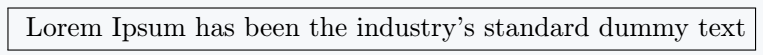2. \framebox[width][position]{text} command creates a visible LR box whose width is determined by the dimension assigned to the width which is our first optional argument. The position of the text is given by the second optional argument. If the position optional argument is neglected, the text is by default centered.

\documentclass{article}
\begin{document}
$$\framebox{Welcome to \LaTeX{}}$$
$$\framebox[5cm][l]{Justify text to left}$$
$$\framebox[5cm][r]{Justify text to right}$$
$$\framebox[5cm]{Justify text to center}$$
$$\framebox[5cm][c]{Justify text to center}$$
$$\framebox[5cm][s]{Justify text to left}$$
\end{document}

Output :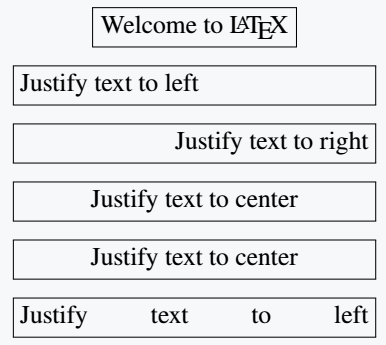The different position arguments that \framebox[width][position]{text} takes include:3. \mbox{text} command creates an invisible LR box with same width as that of text within the brace. text within the brace is not framed. E.g

\documentclass{article}
\begin{document}
\mbox{Lorem Ipsum has been the industry's standard dummy text}
\end{document}

Output :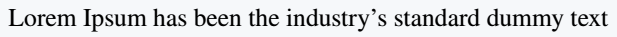4. \makebox[width][position]{text} command creates an invisible LR box whose width is determined by the dimension assigned to the width which is our first optional argument. The position of text is given by second optional argument. If the position optional argument is neglected, the text is by default centered.

\documentclass{article}
\begin{document}
$$\makebox{Welcome to \LaTeX{}}$$
$$\makebox[5cm][l]{Justify text to left}$$
$$\makebox[5cm][r]{Justify text to right}$$
$$\makebox[5cm]{Justify text to center}$$
$$\makebox[5cm][c]{Justify text to center}$$
$$\makebox[5cm][s]{Justify text to left}$$ $$\end{document} Output :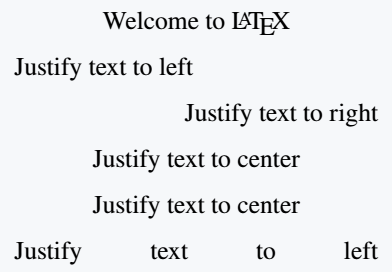The different position arguments that \framebox[width][position]{text} take include:Summarily,LaTeX also gives a possibility of defining width of an LR box relative to its lengths produced by a simple command also known as natural dimensions. They are actually 4 of these length parameters base on a clear demonstration.They are \width, \height, \depth, and \totalheight = \height + \depth \documentclass{article} \begin{document}$$\framebox[1.5\width]{Lorem Ipsum is not simply random text}\framebox[40\height]{Lorem Ipsum is not simply random text}\framebox[100\depth]{Lorem Ipsum is not simply random text}\framebox[30\totalheight]{Lorem Ipsum is not simply random text}
\end{document}

Output :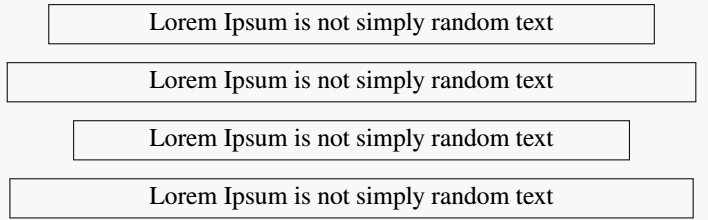These length parameters will only work within the width specification of an LR box, or within the height specification of a paragraph box which we will address in another tutorial. In any other context, they will produce an error message.

## Single line text box style

There are two parameters responsible for styling frame boxes \fbox and \framebox. These parameters are:

• \fboxrule determines thickness of the frame lines,
• \fboxsep sets the amount of spacing(distance) between frame and text covered by frame. The default distance is 3pt.

These two parameters can be defined as follows within your work.

\documentclass{article}
\usepackage{amsmath}
\begin{document}
\setlength{\fboxrule}{0.5em}
\setlength{\fboxsep}{2em}
\fbox{The quick brown fox jumps over the lazy dog}
\end{document}

Output :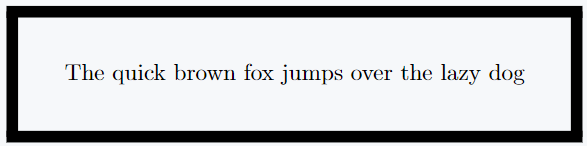We saw how to use the \setlength command in the Lengths and units tutorial. The above code does the following:

• sets the thickness of the frame line to 0.5em
• sets the distance(spacing) between the frame and the text to 2em.

When this length is defined as seen above within the body of work in between curly braces { }, the effect will only be seen on the frame with the braces but if the parameters are defined as lengths in the preamble, then they affect every frame box in the entire document. i.e

\documentclass{article}
\usepackage{amsmath}
\setlength{\fboxrule}{0.5em}
\setlength{\fboxsep}{2em}
\begin{document}
\fbox{The quick brown fox jumps over the lazy dog}
\end{document}

Output :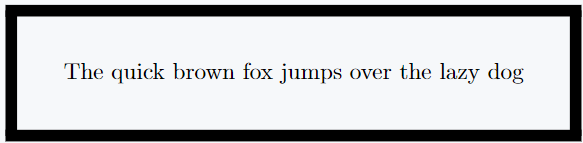## Fancybox Frames

The Fancybox usage package provides us has four types of LaTeX \fbox commands for framing text(elements) in LaTeX. They include:

1. \shadowbox{Lorem Ipsum is not simply random text}Added to the two box styling parameters above, \shadowbox has a third parameter that regulate the width of the shadow called \shadowsize with default 4pt. For example

\documentclass{article}
\usepackage{amsmath}
\usepackage{fancybox}
\begin{document}
\setlength{\fboxrule}{0.5em}
\setlength{\fboxsep}{2em}
\shadowbox{The quick brown fox jumps over the lazy dog}
\end{document}

Output :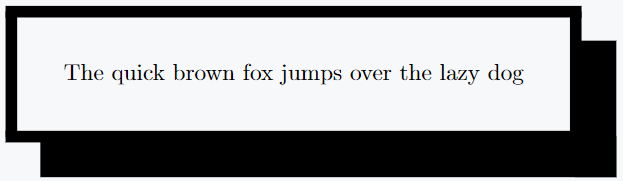2.\doublebox: The width of the inner frame is 0.75\fboxrule, and the width of the outer frame is 1.5\fboxrule. The distance between the two frames is 1.5\fboxrule plus 0.5pt. For example

\documentclass{article}
\usepackage{amsmath}
\usepackage{fancybox}
\begin{document}
\doublebox{The quick brown fox jumps over the lazy dog}\\[1em]
{\setlength{\fboxrule}{0.5em}
\setlength{\fboxsep}{1em}
\doublebox{The quick brown fox jumps over the lazy dog}
\end{document}

Output :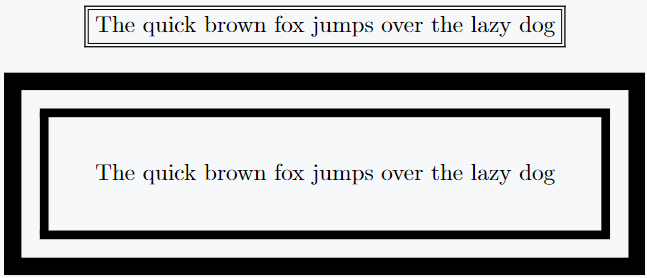3.\ovalbox and \Ovalbox : \ovalbox and \Ovalbox command generates a frame with rounded corners. The width of the frame corresponds to the \thinlines declaration and the \Ovalbox command frame width corresponds to the size produced by a \thicklines declaration.

The diameter of the corner arcs is set with a \cornersize declaration. The command \cornersize{num} sets the diameter to num × minimum (width of box, height of box);
The command \cornersize*{length} sets the diameter to the length in the brace. The default is \cornersize{0.5}.

\documentclass{article}
\usepackage{amsmath}
\usepackage{fancybox}
\begin{document}
\ovalbox{The quick brown fox jumps over the lazy dog}\\[1em]
\Ovalbox{The quick brown fox jumps over the lazy dog}\\[1em]
{
\setlength{\fboxrule}{1.5em}
\setlength{\fboxsep}{1em}
\cornersize{2}
\ovalbox{The quick brown fox jumps over the lazy dog}\\[1em]
\cornersize*{3cm}
\Ovalbox{The quick brown fox jumps over the lazy dog}
}
\end{document}

Output :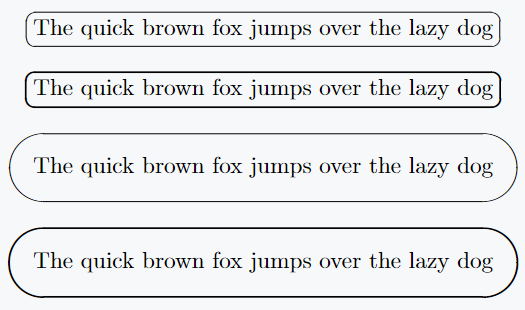The shadow package provides us with the \shabox command which is very similar to the LaTeX command \fbox, except that a “shadow” is added to the bottom and the right side of the box.

The package gives us 3 control parameters: \sboxrule defines the width of the lines for the frame (0.4pt); \sboxsep which defines the separation between the frame and the text. By default, it is 10pt; and \sdim specifies the dimension of the shadow. By default it is 4pt. For example

\documentclass{article}
\usepackage{amsmath}
\begin{document}
\setlength{\sboxrule}{0.5em}
\setlength{\sboxsep}{1.5em}
\setlength{\sdim}{7pt}
\shabox{The quick brown fox jumps over the lazy dog}
\end{document}

Output :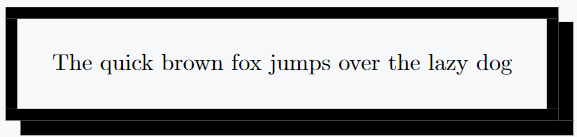## Nesting Boxes

The box commands discussed above call me nested as we want without any error. Below we have few examples. We will revisit box nesting in paragraph box tutorials since paragraphs can be inserted into single line(LR) boxes and at the same time the boxes can be nested.

\documentclass{article}
\usepackage{amsmath}
\begin{document}
\setlength{\fboxrule}{0.25em}
\setlength{\fboxsep}{1em}
\fbox{\fbox{The quick brown fox jumps over the lazy dog}}
\end{document}

Output :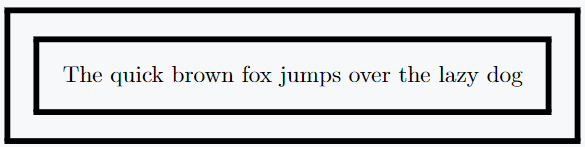\documentclass{article}
\usepackage{amsmath}
\usepackage{fancybox}
\begin{document}
{
\setlength{\fboxrule}{0.25em}
\setlength{\fboxsep}{1em}
\fbox{\Ovalbox{The quick brown fox jumps over the lazy dog}}
}
{
\setlength{\fboxrule}{0.25em}
\setlength{\fboxsep}{0.5em}
\fbox{\fbox{\Ovalbox{The quick brown fox jumps over the lazy dog}}}
}
\end{document}

Output :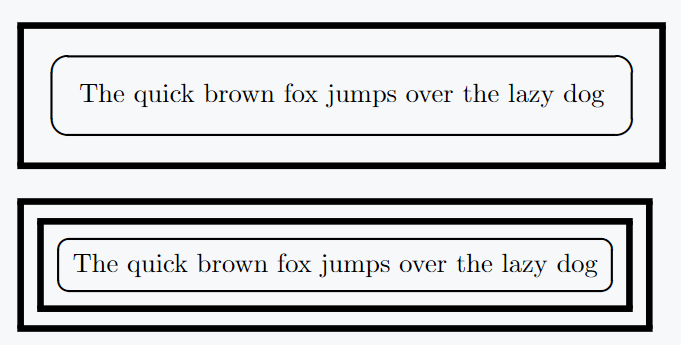## Conclusion

This article gives you a full coverage of how to create the different types of LR and fancy text boxes. It gives you a solid foundation for beautifully presenting ideas in frames and equally will serve as a good foundation for presenting paragraph of text as we will be seeing in our future tutorial on paragraph boxes. A good mastery of this tutorial will set you ready for writing good presentations.